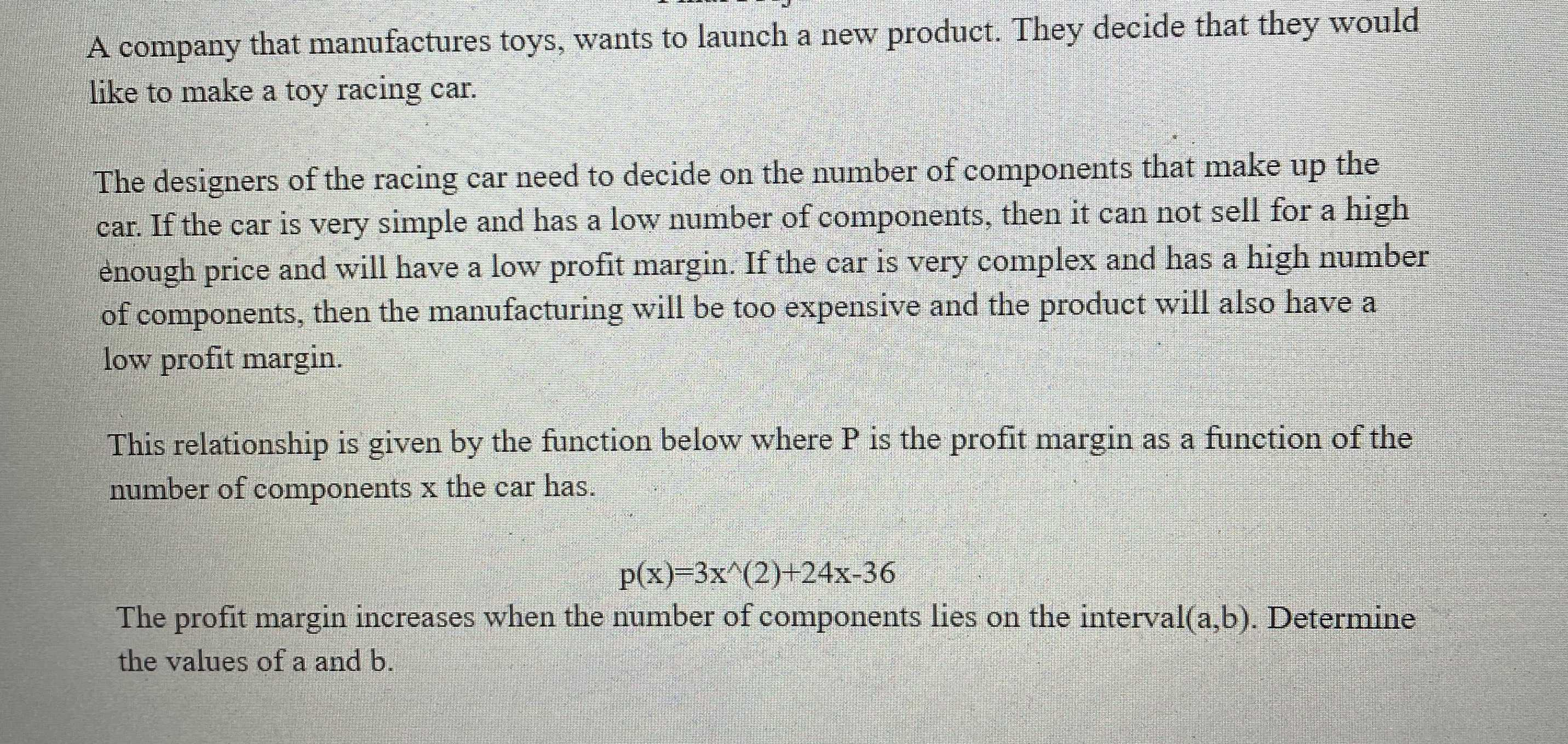### ¿Todavía tienes preguntas de matemáticas?

Pregunte a nuestros tutores expertos
Algebra
PreguntaA company that manufactures toys, wants to launch a new product. They decide that they would like to make a toy racing car. The designers of the racing car need to decide on the number of components that make up the car. If the car is very simple and has a low number of components, then it can not sell for a high enough price and will have a low profit margin. If the car is very complex and has a high number of components, then the manufacturing will be too expensive and the product will also have a low profit margin. This relationship is given by the function below where $$P$$ is the profit margin as a function of the number of components $$x$$ the car has. The profit margin increases when the number of components lies on the interval(a,b). Determine the values of a and $$b$$ .

$$(- 4,\infty)$$ as function is increasing in this interval.